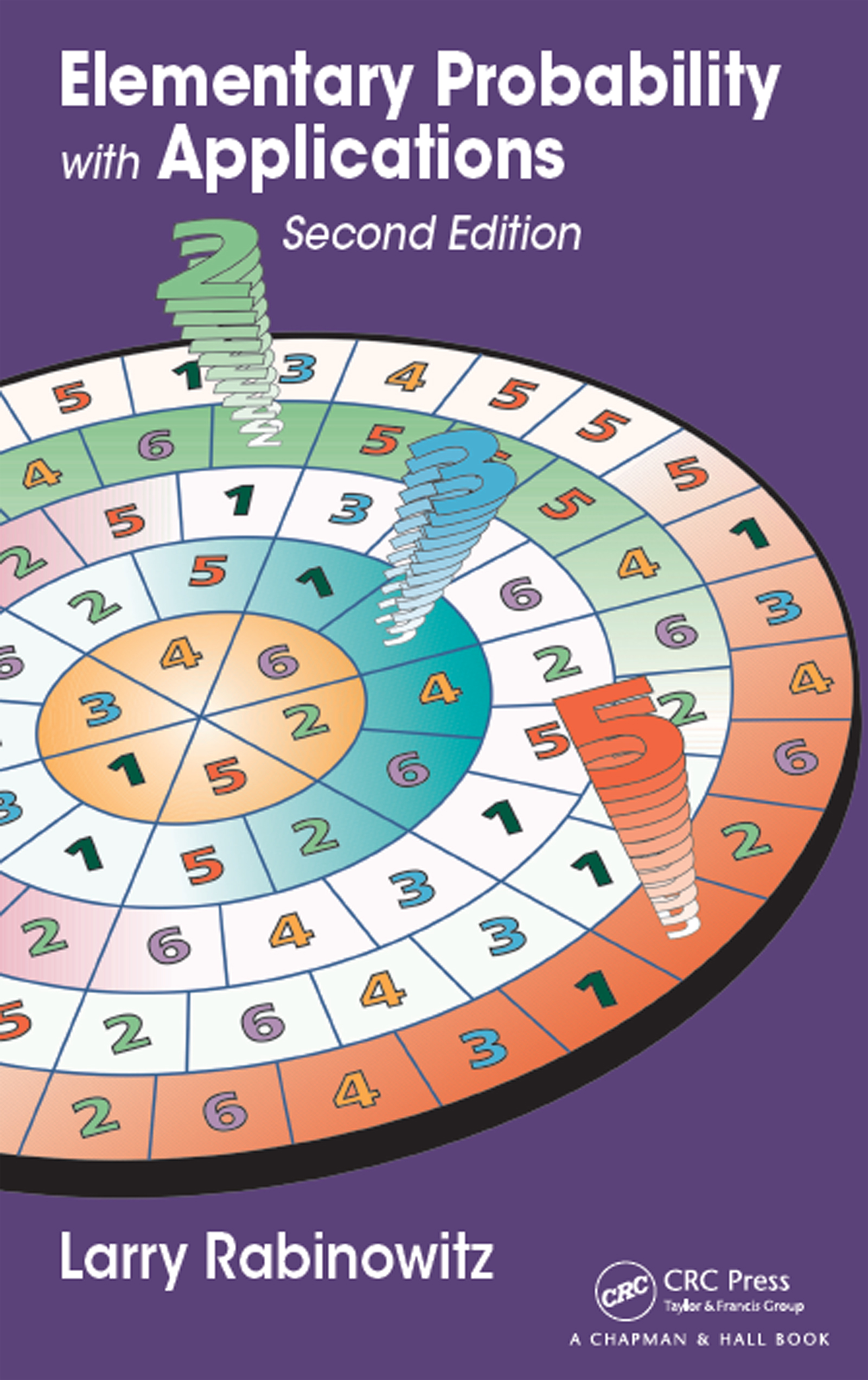# Elementary Probability with Applications

## 2nd Edition

Chapman and Hall/CRC

208 pages | 11 B/W Illus.

Hardback: 9781498771320
pub: 2016-12-13
SAVE ~\$14.99
\$74.95
\$59.96
x
eBook (VitalSource) : 9781315367149
pub: 2016-11-03
from \$37.48

FREE Standard Shipping!

### Description

Elementary Probability with Applications, Second Edition shows students how probability has practical uses in many different fields, such as business, politics, and sports. In the book, students learn about probability concepts from real-world examples rather than theory.

The text explains how probability models with underlying assumptions are used to model actual situations. It contains examples of probability models as they relate to:

• Bloc voting
• Population genetics
• Doubling strategies in casinos
• Machine reliability
• Airline management
• Cryptology
• Blood testing
• Dogs resembling owners
• Drug detection
• Jury verdicts
• Coincidences
• Number of concert hall aisles
• 2000 U.S. presidential election
• Points after deuce in tennis
• Tests regarding intelligent dogs
• Music composition

Based on the author’s course at The College of William and Mary, the text can be used in a one-semester or one-quarter course in discrete probability with a strong emphasis on applications. By studying the book, students will appreciate the subject of probability and its applications and develop their problem-solving and reasoning skills.

### Reviews

Praise for the First Edition:

"It is desirable that all citizens have an elementary understanding of probability [so] that they better appreciate the uncertainty that surrounds them. Anyone teaching such citizens should consider using this book because, through its applications, it conveys something of the power of probability."

—D.V. Lindley, Mathematical Gazette, November 2006

"This book was surprisingly refreshing and did a great job using real situations to motivate techniques for calculating discrete probabilities."

Technometrics, August 2006

"The problems are graded from fairly straightforward to very challenging and the book is, for this reason at least, a welcome addition to the literature."

MAA Reviews, October 2005

"This book would be excellent for a minicourse at a higher level of high school as well as a semester course at the college level."

—Larry White, National Council of Teachers of Mathematics (NCTM), October 2005

"The chosen approach is practical and entertaining. The book is a useful tool for teachers and anybody interested in basic ideas and applications of classical probability theory."

Basic Concepts in Probability

Sample Spaces, Events, and Probabilities

Simulations

Complementary Events and Mutually Exclusive Events

Some Probability Rules

Problem Solving

Problems

Conditional Probability and the Multiplication Rule

Conditional Probability

Multiplication Rule

Problems

Independence

Independence

A Technique for Finding P(A or B or C or …)

Problems

Combining the Addition and Multiplication Rules

Combining the Addition and Multiplication Rules

Bayes' Formula

Trees

Problems

Combining the Addition and Multiplication Rules—Applications

Randomized Response Designs

Applications in Cryptology

Hardy–Weinberg Principle

Problems

Random Variables, Distributions, and Expected Values

Random Variables, Distributions, and Expected Values

Problems

Joint Distributions and Conditional Expectations

Joint Distributions

Independent Random Variables

Conditional Distributions

Conditional Expectations

Problems

Sampling without Replacement

Counting Formula

Probabilities for Sampling without Replacement

Problems

Sampling with Replacement

Binomial Model

Problems

Sampling with Replacement (Continued)

Binomial Model (Continued)

Problems

Binomial Tests

Introduction

Binomial Tests

Problems

Appendix

Bibliography

Index